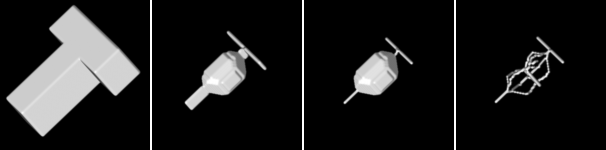# Skeleton types¶

This examples shows various skeleton types available in pi2. Skeletonization is a process where a binary image is converted to single pixel thick lines or planes that still represent the geometry (and perhaps topology) of the object in the original binary image.

Pi2 supports three types of skeletons.

• The command surfaceskeleton with default arguments creates skeletons that consist of 1-pixel thick planes and in some cases the planes may degenerate into 1-pixel thick lines.
• The command surfaceskeleton with ‘retain surfaces’ argument set to false creates skeletons that consist of 1-pixel thick lines where possible. Cavities in the original binary image are still surrounded by a 1-pixel thick plane.
• The command lineskeleton creates skeletons that consist strictly of 1-pixel thick lines.

Note that skeletons calculated in distributed processing mode might not be equal to skeletons calculated in normal processing mode. In both cases the skeletons should be correct, though.From left to right: Slice of the input image, slice of a surface skeleton, slice of a surface skeleton with ‘retain surfaces’ set to false, slice of a line skeleton.From left to right: Visualization of the input image, visualization of a surface skeleton, visualization of a surface skeleton with ‘retain surfaces’ set to false, visualization of a line skeleton.

Code used to create image data for the figures above:

def skeleton_types():
"""
Demonstrates differences between skeletonization algorithms available in pi2.
"""

# Create a 3D image that contains a thick T letter
img = pi.newimage(ImageDataType.UINT8, 100, 100, 100)
pi.box(img, [20, 20, 40], [61, 21, 26], 255)
pi.box(img, [40, 20, 40], [21, 71, 26], 255)

# Add a cavity (hole) to the T
pi.sphere(img, [50, 50, 50], 5, 0)

# Save the input geometry
pi.writeraw(img, output_file('T'))

# Create a skeleton that contains 1-pixel thick planes and 1-pixel thick lines.
surface_skele_true = pi.newlike(img)
pi.set(surface_skele_true, img)
pi.surfaceskeleton(surface_skele_true, True)
pi.writeraw(surface_skele_true, output_file('T_surface_skele_true'))

# Create a skeleton that contains 1-pixel thick planes only around cavities
# and 1-pixel thick lines elsewhere.
surface_skele_false = pi.newlike(img)
pi.set(surface_skele_false, img)
pi.surfaceskeleton(surface_skele_false, False)
pi.writeraw(surface_skele_false, output_file('T_surface_skele_false'))

# Create a skeleton that contains only 1-pixel thick lines.
line_skele = pi.newlike(img)
pi.set(line_skele, img)
pi.lineskeleton(line_skele)
pi.writeraw(line_skele, output_file('T_line_skele'))